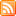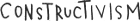# Non-regular Parameters are OK

Both Coq and Agda let the user declare datatypes with non-regular parameters which do vary, are not quite indices and can be large without bumping the universe level in which the declaration lives. In his habilitation thesis, Bruno Barras shows a nice trick explaining why these non-regular parameters make sense.

### # Quick definition

A non-regular parameter for an inductive type is an (usually large) argument of the type constructor which is used uniformly in the return type of its term constructors (thus making it parameter-like) but may vary in their recursive positions (making it index-like).

First of all, let's see a couple of use cases for non-regular parameters. In Ralf Hinze and Ross Paterson's fingertrees, the non regular parameter « determines the unusual shape of these trees, which is the key to their performance » . The non regularity is here used to enforce invariants in the substructures by tightly controlling which constructors one may use.

data FingerTree a = Empty | Single a | Deep (Digit a) (FingerTree (Node a)) (Digit a)

Using Type-level de Bruijn indices in order to bake in a syntax a notion of scope and binding sites is another use of non regularity. In this case, the invariant we are enforcing is the fact that one can only talk about variables which are in scope. But it also formalizes lets us identify clearly the constructors which are binders.

Now that we have seen that this non-regularity can be useful, we would like to know whether it is a safe feature. After all, having a system in which Type : Type can simplify things quite a lot but it comes at the cost of being inconsistent...

### # The encoding

We will not give a generic account of this transformation but explain it by a simple example. Let's use the type-level de Bruijn indices case to demonstrate how one can explain the use of a non-regular parameter in terms of more basic concepts which are known to be sound. We will work in Agda but it would be possible to perform the same construction in Coq as demonstrated by this file.

#### # What one would write in Agda

We start by defining our simple lambda calculus Lam equipped with a let binder. The parameter A represents the variables available in scope; it needs therefore to be modified every time a binder introduces fresh ones :

data Lam (A : Set) : Set where `var : (a : A) → Lam A `app : (t u : Lam A) → Lam A `lam : (t : Lam (1 + A)) → Lam A `let_`in_ : {n : ℕ} (t : Vec (Lam A) n) (u : Lam (n + A)) → Lam A

#### # A naïve translation using large indexes

Given that this argument varies in the various recursive positions, one could consider calling it an index. This is where we run into troubles: the value A used to be in context because it was treated like a parameter; now that we consider it to be an index, every constructor has to introduce A to be able to mention it. But A is large which forces the datatype declaration to live one level up.

data Lam : (A : Set) → Set₁ where `var : {A : Set} (a : A) → Lam A `app : {A : Set} (t u : Lam A) → Lam A `lam : {A : Set} (t : Lam (1 + A)) → Lam A `let_`in_ : {A : Set} {n : ℕ} (t : Vec (Lam A) n) (u : Lam (n + A)) → Lam A

This is quite clearly not a satisfactory solution. It is, for instance, now impossible to define the monadic join for Lam because Lam (Lam A) is not even a type one can form! If we do need to introduce an index to track down the modifications made to the non regular parameter then it'd better be a small one. This is precisely what the encoding does.

#### # Barras' encoding

The important remark behind this encoding is that the shape of the non-regular parameter at any point in a tree is entirely determined by the value of the parameter at the root; the path followed from the root down to this subtree and, potentially, additional information stored in the nodes encountered along this path. Now, this information has to be small, otherwise the inductive definition would not fit at its current level. It is therefore sufficient to store, as a parameter, the value present at the root and then use a small index to describe the path followed and the information accumulated. In the case of our running example, the type of path is as follows.

data path : Set where `rt : path `bd : (n : ℕ) (p : path) → path

`rt represents the root of the tree and `bd (for "binder") represents both `lam and `let_`in_ because we are only interested in the number of variables introduced by a binding site. We note that one can blithely forget about the `app constructors one crossed because they do not alter the parameter. The decode function defining the semantics of these paths is straightforward: the root induces the identity and a binding site extends the environment with n new variables.

decode : (p : path) (A : Set) → Set decode `rt A = A decode (`bd n p) A = n + decode A p

This allows us to define a datatype in a theory with only parameters and indices whilst keeping it small. As one would expect, it is possible to prove that this inductive type is in bijection with the original one. The usual problem arise with the termination checker when dealing with the nesting Vec (Lam A) n but, as usual, inlining Vec.map is sufficient to convince Agda that everything is fine.

data Lam (A : Set) : path → Set where `var : {p : path} (a : decode p A) → Lam A p `app : {p : path} (t u : Lam A p) → Lam A p `lam : {p : path} (t : Lam A (`bd 1 p)) → Lam A p `let_`in_ : {p : path} {n : ℕ} (t : Vec (Lam A p) n) (u : Lam A (`bd n p)) → Lam A p

### # Conclusion

Well that was fun! If you want a more general presentation, then have a look at the thesis page 138 and following. Beware though: I started reading it because it was mentioned somewhere that it explained how to make sense, in terms of indexed families, of mutually defined inductive types leaving at different levels; and stayed because I kept seeing other interesting topics. It's a trap.

### # Footnotes

Page 4, Finger trees: a simple general-purpose data structure by Ralf Hinze, Ross Paterson.

_+_ of type ℕ → Set → Set is used here to mean that a finite number of new bound variable have been introduced by the binding construct.

Last update: 2021 11